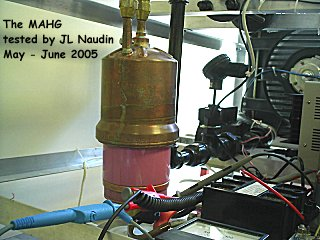Free Energy from Atomic Hydrogen
MAHG - LONG TEST RUNS
Created on June 2, 2005 - Updated on June 2, 2005

The purpose of these tests is to conduct some LONG TEST RUNS on the MAHG. So, I have conducted 3 RUNS of different durations : 30 min, 1 hour, and 2 hours at FULL POWER.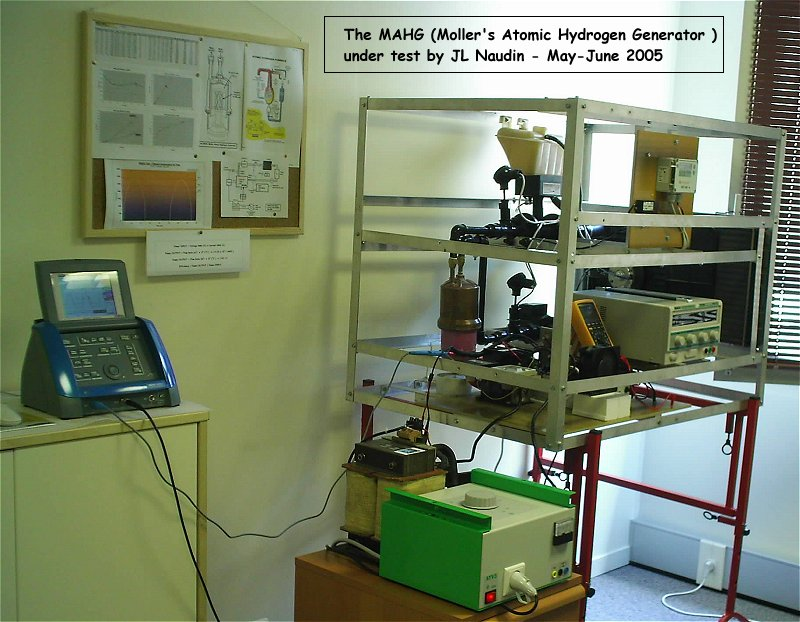The MAHG under the long tests runs (DC pulsed mode)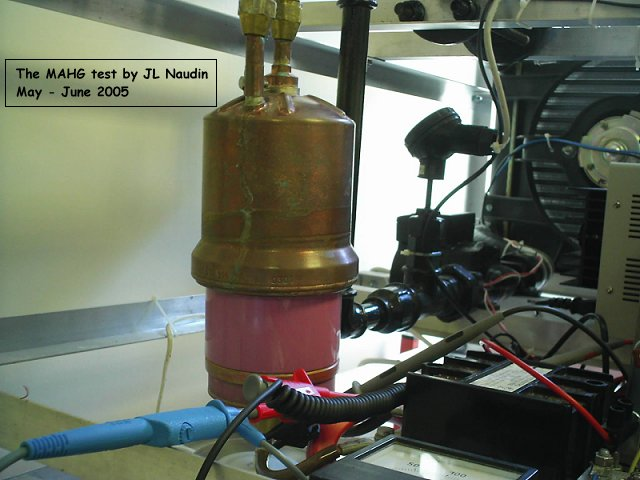During these tests the pink area (the dielectric ring) of the reactor becomes hot (>60°C).
There are a lot of losses (~ 30%) through this part (see the blank tests). In next tests, this area will be insulated to prevent thermal losses.

Test procedure :

• 1) Before each RUN, the MAHG device is cooled with the fan for at least 30 min so as to get a stable Input/Output temperature.

• 2) The initial conditions are carefully noted : the initial water flow (S_F_m3/h), the inital temperature (Ts2) at the input and at the output (Ts1) and the temperature correction value (T Offset = Ts1 - Ts2) for the initial conditions is calculated.

• 3) At the end of the long RUN the final conditions are noted : the final water flow (F_F_m3/h), the final temperature (Tf2) at the input and at the output (Tf1) and the temperature difference (dT = (Tf1 - Tf2) - (T Offset)) is calculated. The average voltage and current are also measured on the digital oscilloscope.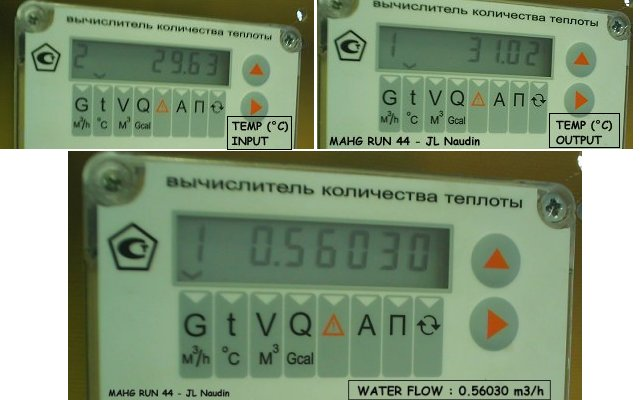A close view of the datas collected on the BKT-4M computer

Data sheet results details :

• Input (V), Input (A) : Rms Voltage and Rms Current at the input

• Mode : Mono Alt, single DC pulse mode (half sine wave) at 50% of duty cycle

• S_F_m3/h : Water flow measured at the start of the run in m3/h

• F_F_m3/h : Water flow measured at the end of the run in m3/h

• AVG_F : Average flow of water during all the run in m3/h

• Ts1 : Output temperature of the water at the start of the run

• Ts2 : Input temperature of the water at the start of the run

• T Offset = Ts1 - Ts2 : temperature correction value for the initial conditions

• Tf1 : Output temperature of the water at the end of the run

• Tf2 : Input temperature of the water at the end of the run

• dT : Temperature difference at the end of the run, dT = (Tf1 - Tf2) - (T Offset)

• PWR INP : Electrical power at the input

• PWR OUT : Thermal power at the output :

• Power OUTPUT = Flow Rate (m3/h) x dT (°C ) x ( 4,18 x 106 / 3600 ) = Flow Rate (m3/h) x dT (°C ) x 1161,11

Efficiency = Power OUTPUT / Power INPUT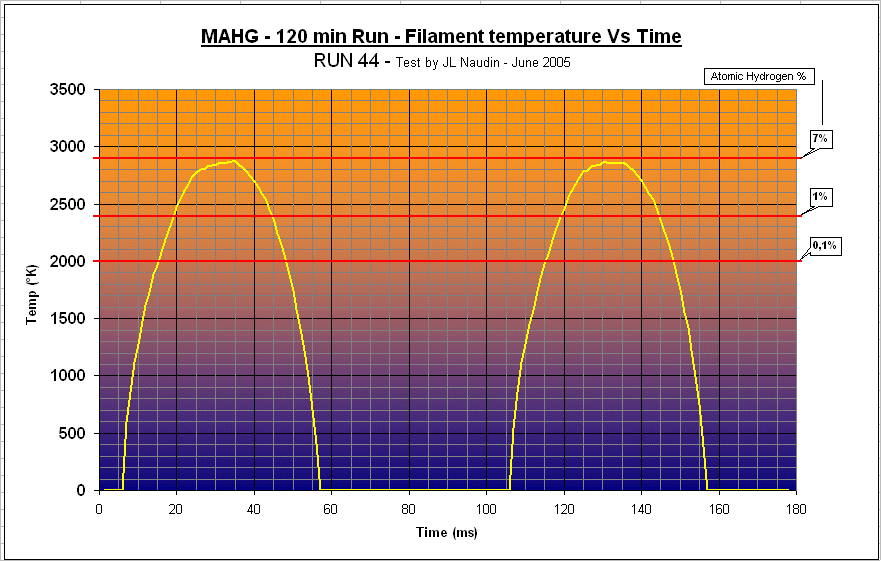The experimental curve above shows the MAHG filament (the cathod) temperature VS time during the tests.
You may notice that atomic hydrogen (1% to 7%) has been produced during only 25 ms each 100 ms.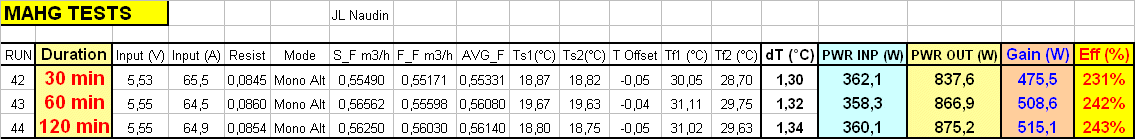Comments from Jean-Louis Naudin : These long test runs on the MAHG are very encouraging because they demonstrate clearly that this device is able to provide a continuous and stable power flow (875 Watts) at its output for a long time (2 hours) with an efficiency of about 240% and with a net power gain of 515 Watts.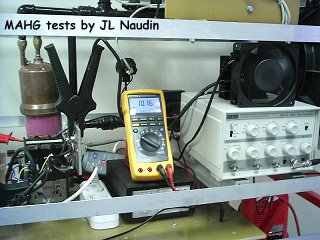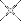Towards HIGH EFFICIENCY ( > 1000% )